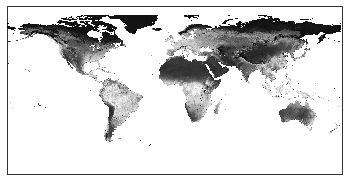# Use the WCS data interface with Python

Important features of Geo Engine are data interfaces, with which users can further use data sources or computations (workflows) in other programs or environments. We also support standard interfaces of the OGC, e.g. the Web Coverage Service (WCS). With this service, raster data can be retrieved in formats that can be further processed and thus easily integrated into data science workflows or GIS tools.

## A machine learning example in a Jupyter Notebook

Geo Engine provides a Python library that makes workflows easily accessible programmatically. Here, we also use the OGC standard interface WCS to make raster data accessible and processable as numpy arrays.

In this example, we ingest NDVI data from MODIS¹ into the machine learning framework scikit-learn. NDVI is a vegetation index that allows inferences about the amount and vitality of plants. Here, we use Geo Engine for temporal data access, where we integrate different time steps of the dataset into our process.

Initially, we import the geoengine package as well as scikit-learn into our Jupyter Notebook environment.

```from sklearn.cluster import KMeans
from datetime import datetime
import matplotlib.pyplot as plt

import geoengine as ge
```

We then initiate the connection to a Geo Engine instance.

```ge.initialize("http://localhost:3030")
ge.get_session()
```
```Server:              http://localhost:3030
Session Id:          4565bdfa-956e-4dcf-8be6-56c6b02ec1b5
```

We register the NDVI workflow and get back a workflow object on which we can start the data access in the following.

```workflow = ge.register_workflow({
"type": "Raster",
"operator": {
"type": "GdalSource",
"params": {
"dataset": {
"type": "internal",
"datasetId": "36574dc3-560a-4b09-9d22-d5945f2b8093"
}
}
}
})
workflow
```
```8df9b0e6-e4b4-586e-90a3-6cf0f08c4e62
```

First, we want to get an overview of the data and start a WMS access for April 2014, which returns us a geo-referenced image.

```time = datetime.strptime(
'2014-04-01T12:00:00.000Z', "%Y-%m-%dT%H:%M:%S.%f%z")

workflow.plot_image(
ge.QueryRectangle(
[-180.0, -90.0, 180.0, 90.0],
[time, time]
)
);
```We can see different shades of NDVI values, where in this case lighter means more vegetation. Thus, there are different areas that we can divide into classes depending on the degree of vegetation.

To be able to use the raw data for machine learning, from now on we request the data using WCS. In our Python library, this is done with the get_array function. The returned data is a numpy array, which is the de-facto standard in Python for handling array data.

```width = 128

time = datetime.strptime(
'2014-04-01T12:00:00.000Z', "%Y-%m-%dT%H:%M:%S.%f%z")

wcs_array = workflow.get_array(
ge.QueryRectangle(
[-180.0, -90.0, 180.0, 90.0],
[time, time],
resolution=[360./width, 180./width],
)
)

plt.imshow(wcs_array)
```

Here we have requested and plotted the data with a resolution of 128×128 pixels. For the partitioning into classes, we use the clustering algorithm k-Means, which can perform a simple partitioning for k classes based on their values. For training, we continue to use the month of April 2014 and consider results for multiple numbers of classes k.

```fig, axes = plt.subplots(2, 2, figsize=(10, 10))
axes = [ax for row in axes for ax in row]

for k, ax in zip(range(2, 6), axes):
kmeans = KMeans(n_clusters=k, random_state=0).fit(wcs_array.reshape(width**2, 1))

ax.imshow(kmeans.labels_.reshape(width, width))
ax.set_title(f'k = {k}')

fig.suptitle('k-Means Clustering Results')
fig.tight_layout()

plt.show()
```

We recognize several segmentations that are roughly similar to our initial assessment of the dataset. There are differences between water areas (no vegation), little vegetation (North Africa) and much vegetation (East Asia). In this example, we decide to divide the data into four classes (k=4) and want to continue with this by dividing the other time points into classes accordingly.

For this we start the same data access using Geo Engine, but we change the requested time by one month each step. This means that we train a model for April 2014 and four classes and apply it for different time steps each time. This is done in the following with a loop.

```k = 4
kmeans = KMeans(n_clusters=k, random_state=0).fit(wcs_array.reshape(width**2, 1))

fig, axes = plt.subplots(3, 2, figsize=(10, 15))
axes = [ax for row in axes for ax in row]

for month, ax in zip(range(1, 7), axes):
time = datetime.strptime(f'2014-{month:02d}-01T12:00:00.000Z', "%Y-%m-%dT%H:%M:%S.%f%z")

wcs_array = workflow.get_array(
ge.QueryRectangle(
[-180.0, -90.0, 180.0, 90.0],
[time, time],
resolution=[360./width, 180./width],
)
)

ax.imshow(
kmeans.predict(wcs_array.reshape(width**2, 1)).reshape(width, width)
)
ax.set_title(time.date())

fig.suptitle('4-Means Predictions')
fig.tight_layout()

plt.show()
```

The shift of classes over time is clearly visible. This is noticeable, for instance, in Central Asia and Europe.

## Summary

Using Geo Engine it is easy to get data into a programming environment like Jupyter Notebooks via standard interfaces and use it in machine learning workflows. In this process, our Python library provides very easy access that also works in the same way in other GIS programs. This process integration is very important to us, as geospatial data processing and analysis can be a multi-layered process until the desired result is obtained.

This use case demonstrates a number of features of Geo Engine:

• The data access of raster data
• The utilization of the OGC standard interface WCS
• The use of the Geo Engine Python library
• The integration of Geo Engine worfklows into machine learning processes

﻿

### Data Citation

1. MODIS Vegetation Index Products (NDVI and EVI): https://modis.gsfc.nasa.gov/data/dataprod/mod13.php

﻿